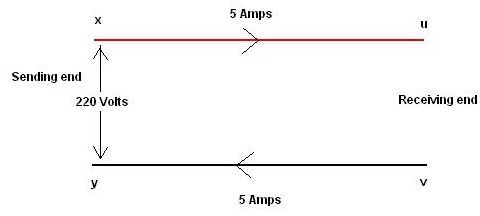# Learn about electricity transmission efficiency and how it is calculated

## Introduction

We know that transmitting electrical power over long distances does involve some amount of losses. This gives rise to the concept of efficiency in transmission and like any other situation; the efficiency just gives an idea about the amount of useful energy which reaches the other side compared to the amount of energy which was fed at one end. So let us see how electrical transmission efficiency is defined and calculated.

## Transmission Efficiency

The general formula for efficiency applies to this case also and

Efficiency of transmission = Po/Pi * 100

Po is the amount of power reaching the receiving end

Pi is the amount of power fed at the sending end

So say for example that 1000 watts of power were fed to a feeder and 900 watts is received at the other end then the efficiency of transmission is 90%. This description will become clearer if you see the derivation of this formula and the diagram given alongside it.As you can see in the picture it shows 2 feeder wires where the end x, y is the sending side while the end u, v is the receiving side. The voltage and current of the power being sent are 220V and 5A respectively.

Let us assume the resistance of each feeder wire to be 1 ohm for simplicity

This means that the drop in potential over one feeder wire would be I * R = 5 Volts. Hence the total drop including both feeders will be 10 Volts.

This obviously means that the voltage obtained at the other end would not be 220 but 210 volts only.

Power associated with 220V and 5A = V * I = 1100 watts

Power associated with 210V and 5A = 1050 watts

So efficiency of transmission = 1050/1100 * 100 = 95.4%

Another method of expressing this efficiency is the equation

E = 100 – (IR/Vi * 100)

Where Vi is the input voltage and E is efficiency.

Hence you can see that for efficiency to be maximum the right hand side figure has to be minimum. This in turn means that Vi should be as high as possible hence the need to step up voltage while transmitting.

Another corollary from this equation is that when voltage is high, current is low. This means that the diameter of the conductor required is lesser and this results in material cost savings for the conductor as thinner cables can be used.

## Feeder Vs Distributor

We saw the difference between feeder and distributor in our previous article. You will remember that a feeder does not have any tapping of power midway but only at its end while a distributor is tapped at various places because of its function. Hence the above equation only applies to a feeder setup and not a distributor.